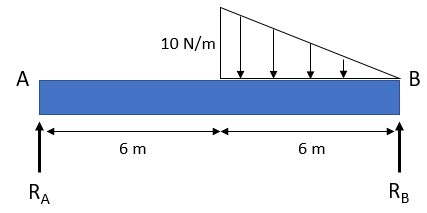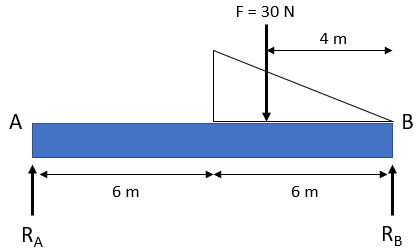$$F=\frac{1}{2}(base)(height)$$$Hint 2 Calculate the sum of moments about Point A to determine the reaction at Point B: $$\sum M_{A}=0$$$
$$F=\frac{1}{2}(base)(height)=\frac{1}{2}(6m)(10\frac{N}{m})=30\:N$$$Next, let's calculate the sum of moments about Point A. We will be able to determine the reaction at Point B: $$\sum M_{A}=0=(force)(distance)$$$
$$R_A(0m)+(12m)R_B-(30N)(8m)=0$$$$$(12m)R_B=(30N)(8m)$$$
$$R_B=\frac{240N\cdot m}{12m}=20\:N$$\$